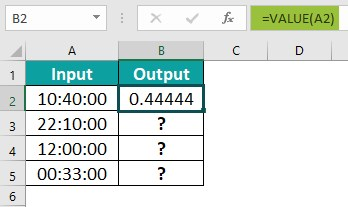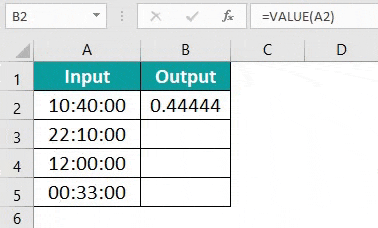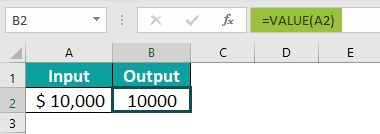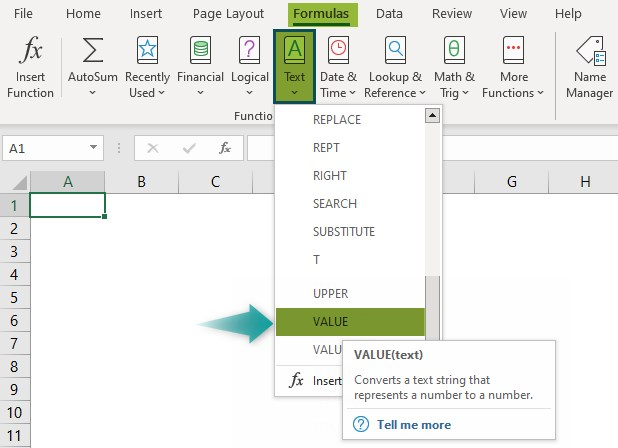# Value Function ExcelArticle byExcelMojo Team## What Is VALUE Function In Excel?

The VALUE Function in Excel converts a text string representing a numeric value into a number. When the text string is in one of the formats, such as date, number, time, etc., we get the “#VALUE” error, which is converted to a numeric value using this function.

The VALUE function in Excel is an inbuilt function, which means that we can insert it as a formula from the “Function Library” or enter it directly in the worksheet. For example, we will apply the VALUE Function in Excel to calculate the negative number value. Select cell B2, enter the formula =VALUE(A2), and press the “Enter” key.

The output is “-10”, as shown above. The value of the selected cell is the negative number itself.

###### Key Takeaways
• The VALUE Function in Excel converts the text strings with formats such as date, time, fractions, percentage, etc… into a numeric value.
• The VALUE function has only one argument, i.e., text. The function will take only one cell reference at a time. The function will give an error if we select multiple cells or a cell range. Therefore, once we enter the formula and get the required result, then we can copy the formula to the other cells.
• This function makes the Excel data compatible with other spreadsheet applications.

### VALUE() Excel Formula

The Syntax of the VALUE function in Excel formula is,

The argument of the VALUE function in Excel formula is,

• text: It is a mandatory argument. The text refers to a cell containing the text we want to convert.

### How To Use The VALUE Excel Function?

We can use the VALUE Excel Function in 2 methods, namely,

1. Access from the Excel Ribbon.
2. Enter in the worksheet manually.

#### Method #1 – Access from the Excel ribbon

First, choose an empty cell for the output > select the “Formulas” tab > go to the “Function Library” group > click the “Text” option drop-down > select the “VALUE” function, as shown below.

The “Function Arguments” window appears. Enter the argument in the “Text” field and click “OK”, as shown below.

#### Method #2 – Enter in the worksheet manually

1. Select an empty cell for the output.
2. Type =VALUE( in the selected cell. [Alternatively, type “=V” and double-click the VALUE function from the list of suggestions shown by Excel.]
3. Enter the arguments as a cell reference in Excel.
4. Click “OK”.
5. Press the “Enter” key.

Let us take an example to understand this function. Here, we will convert the time value to its numeric value using VALUE Function in Excel.

In the table, the data is,

• Column A contains the Input.
• Column B will display the results that get converted to numeric values.

The steps to convert the input values using VALUE Function in Excel are as follows:

1. Select cell B2, enter the formula =VALUE(A2), and press the “Enter” key. The output is 0.4444, as shown below.2. Drag the formula from cell B2 to B5 using the fill handle.The output is shown above. All the time values in Column A are converted to their respective numeric values, as displayed in Column B.

### Examples

We will understand the VALUE function in Excel with some advanced scenarios.

#### #1 Example

We will convert the dates that are in different formats into numeric values using the VALUE function in Excel.

In the table, the data is,

• Column A contains the Input.
• Column B will display the results that get converted to numeric values.

The steps to convert the dates to numeric values using the VALUE Function in Excel are as follows:

Step 1: Select cell B2, enter the formula =VALUE(A2), and press the “Enter” key. The result is ‘36527’, as shown below.

Step 2: Drag the formula from cell B2 to B4 using the fill handle.

The output is shown above. All the date formats in Column A are converted to their respective numeric values as displayed in Column B.

#### #2 Example

We will convert the fraction, scientific, and percentage values into numeric values using the VALUE function in Excel.

In the table, the data is,

• Column A contains the Input.
• Column B will display the results that get converted to numeric values.
• Column C is for our reference.

The steps to convert the values using the VALUE Function in Excel are as follows:

Step 1: Select cell B2, enter the formula =VALUE(A2), and press the “Enter” key. The result is shown below.

Step 2: Drag the formula from cell B2 to B4 using the fill handle.

The output is shown above. The fraction, scientific, and percentage values in Column A are converted to their respective numeric values as displayed in Column B.

#### #3 Example

We will convert the values into numeric values and find the difference between the values using the VALUE function in Excel.

In the table, the data is,

• Column A contains the V1.
• Column B contains the V2.
• Column C will display the difference between the converted numeric values.

The steps to convert to numeric values and find the difference using the VALUE Function in Excel are as follows:

Step 1: Select cell C2, enter the formula =VALUE(A2)-VALUE(B2), and press the “Enter” key. The output is ‘-100’, as shown below.

Step 2: Drag the formula from cell C2 to C4 using the fill handle.

The output is shown above. First, all the date formats in Columns A and B are converted to their respective numeric values, and then the difference is calculated as displayed in Column C.

### VALUE Function Returns #VALUE Error

We may get the #VALUE!” error when using the VALUE Function in Excel for the following reasons:

• If the Excel VALUE formula is typed incorrectly.
• The cell references are incorrect or are not in the array.
• A source string appears in a format that does not recognize the VALUE formula.
• A non-numeric value is entered as a parameter.

### Important Things To Note

• The function processes any fractional and numeric values less than or greater than, or equal to zero.
• The text entered that has to be converted into the number must be within double quotes, or else the “#NAME!” error occurs.
• By default, Excel converts the non-numeric values to numbers as required, so we may not use the VALUE function frequently.

1. What does the VALUE function do in Excel?

The VALUE Function in Excel is designed to convert text values to numbers and avoid VALUE errors. The function recognizes numeric data, and other time or date formats. The “text” argument is a text string enclosed in quotation marks or a reference to a cell containing the text to be changed to a number.

The syntax of the VALUE function is as follows =VALUE(text).

2. How do you use the VALUE function in Excel?

The VALUE Function in Excel is used as follows:

#1 – Select an empty cell for the output.
#2 – Type =VALUE( in the selected cell. [Alternatively, type “=V” and double-click the VALUE function from the list of suggestions shown by Excel.]
#3 – Enter the arguments as a cell reference.
#4 – Click “OK”.
#5 – Press the “Enter” key.

For example, we will apply the VALUE function in Excel to calculate the currency number.

Select cell B2, enter the formula =VALUE(A2), and press the “Enter” key.The output is “10000”, as shown above.

3. Where is the VALUE function in Excel?

We can use the VALUE Function in Excel as follows:

First, choose an empty cell for the output – select the “Formulas” tab – go to the “Function Library” group – click the “Text” option drop-down – select the “VALUE” function, as shown below.